## Introduction

We have different number systems like natural numbers, whole numbers, fractions etc.

Why do we need a new number system? Where are integers used? Watch this video to know more about integers.

Now you know the applications of integers. Integers include negative numbers, positive numbers, and zero.Negative integers come in handy when a driver must slow down speed or decelerate. For example, a person is driving a car at the speed of 70 kmph on the highway. After the highway ends, the speed limit drops to 40 kmph, so the driver decelerates by 30 kmph to drive at the correct speed limit.Negative integers are also used to measure depth below sea level. For example, the Dead Sea in Israel is located at approximately 423 m below sea level. This can be represented numerically as –423 m.#### Concepts

The chapter ‘Integers’ covers the following concepts:

#### Operations On Integers

Positive numbers are always greater than negative numbers. For negative numbers, as the digit gets bigger, the number gets smaller.

You know how to add and subtract integers. Let us learn how to simplify expressions involving integers.

You may encounter expressions which have addition and subtraction of integers. Follow these simple steps to solve them.

• Perform operations in parenthesis first.
• Addition and subtraction have the same preference.

Integers are used in many real-life applications. To solve word problems on addition and subtraction of integers, follow these simple strategies.

• Identify the signs of the numbers in the question - Positive or Negative.
• Identify the operation required to be done in the question – Addition or Subtraction.
• Carry out the operation to get the final result.

Mohan makes homemade chocolates and sells them in the neighbourhood. Look at his profit and loss statement for the last 6 months.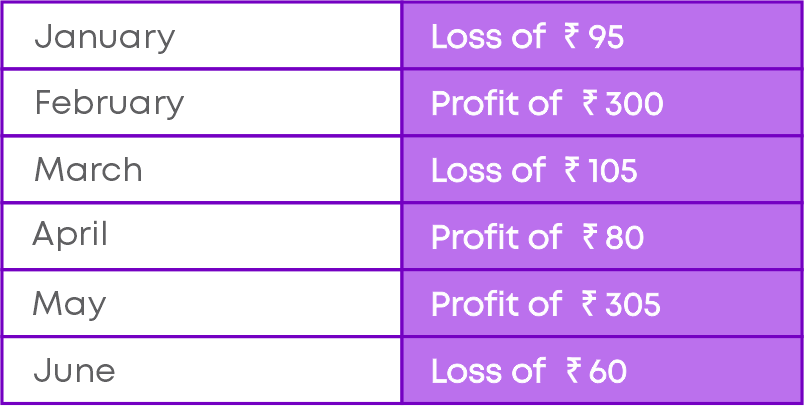Find Mohan’s net profit or loss.

We know that Profit is taken as a positive integer and Loss is taken as a negative integer. To find the net profit/loss, let us add the monthly profit or loss.

Net profit or loss = (-95) + 300 + (-105) + 80 + 305 + (-60)

= 300 + 80 + 305 + (-95 -105 – 60)

= 685 + (-260)

= 685 – 260

= 425.

425 is a positive integer, hence, Mohan has a net profit.

Hence, Mohan has a net profit of ₹425.

Look out for these words that you will find in questions involving integers.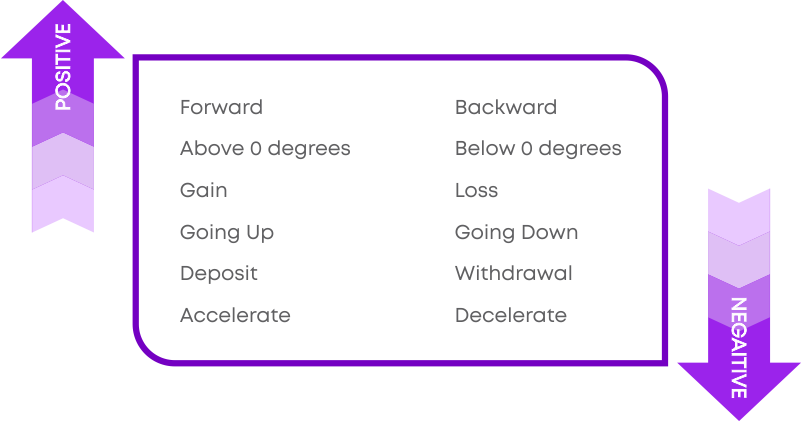The word 'deposit' means

• To put

For example, For my birthday, my father deposited ₹2000 into my account.

The word 'withdraw' means

• To take
• Get reduced
• Withdraw is the opposite of ‘deposit’.

For example, For buying a new dress for my birthday, I have withdrawn an amount of ₹1000 from the bank.

Vertical means,

• Moving from 'top to bottom’ or 'bottom to top'.

For example, When I threw the ball down from the terrace, I noticed that the ball moved vertically down.

Horizontal means

• Moving straight from 'left to right’ or 'right to left'.

Have you noticed that you can slide the touch screen of a smartphone, both horizontally and vertically?Diagonal means

• Slanting

For example, when I climbed the stairs, I found it slanting diagonally.

#### Properties Of Addition And Subtraction Of Integers

You take any two numbers and perform a mathematical operation on them. If the result is also a number from the same type, then the number system is said to be closed for that operation. For example, let us take two natural numbers 2 and 5.

2 + 5 = 7, which is also a natural number.

This is true if you take any two natural numbers. Their sum will always be a natural number.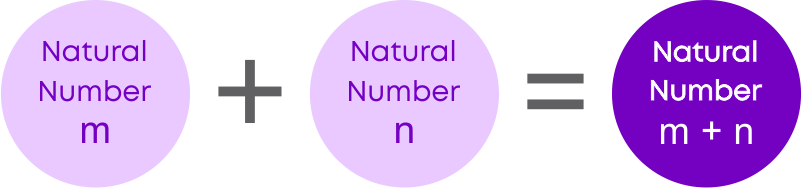Hence, we say natural numbers are closed under addition operation

The sum of two integers is always an integer.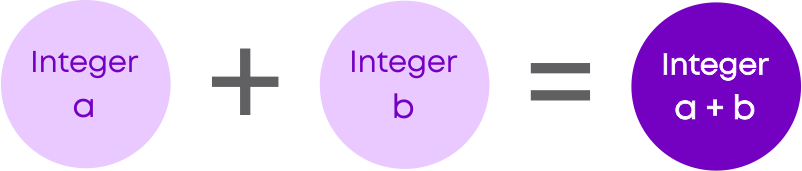The difference of two integers is always an integer.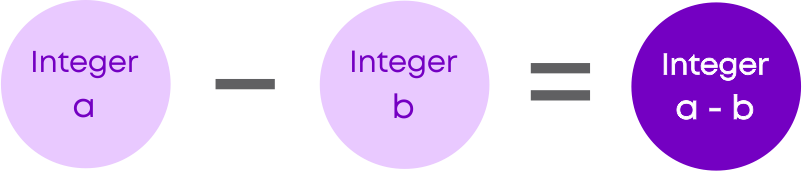Do integers follow commutative property? We will use a number line to understand this by adding the integers (-8) and 5 in two different ways.

On adding 5 to (-8) we get (-3).On adding (-8) to 5, we get (-3).Hence, we found that (-8) + 5 = 5 + (-8).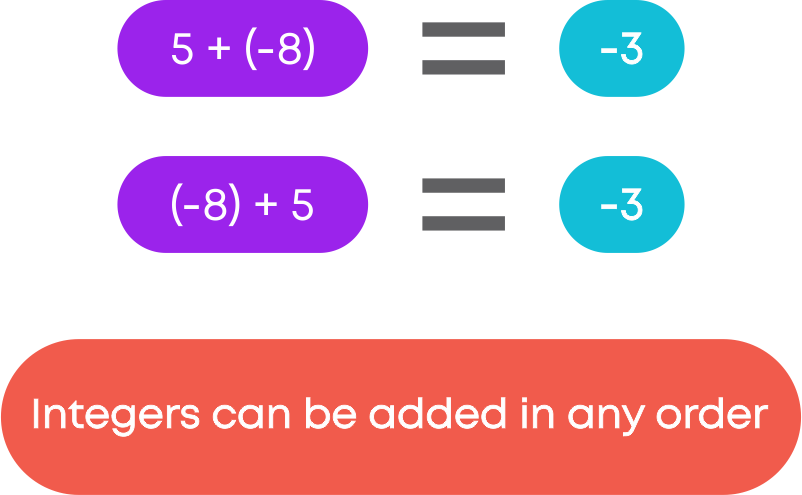Can we subtract two integers in different order and still get the same result? We use a number line to subtract the integers (-8) and 5 in two different ways.

On subtracting 5 from (-8) we get (-13).On subtracting (-8) from 5, we get 13.Hence, we found that (-8) - 5 ≠ 5 - (-8).

So, we can say that subtraction of integers is not commutative.

To add three integers (-8), (-5) and 10, which two integers should be grouped to add first? Are there any rules?The sum of the integers remains the same even though the integers are grouped differently.

In general, if ‘a’, ‘b’ and ‘c’ are three integers then,

(a + b) + c = a + (b + c).

When we add 0 to any integer, we get the same integer as the result. In general, for any integer ‘a’,

a + 0 = a = 0 + a

#### Multiplication Of Integers

You know how to perform multiplication of whole numbers. Let us now see how to perform multiplication of integers. Depending on the signs of the integers that we multiply, the sign of the product also changes.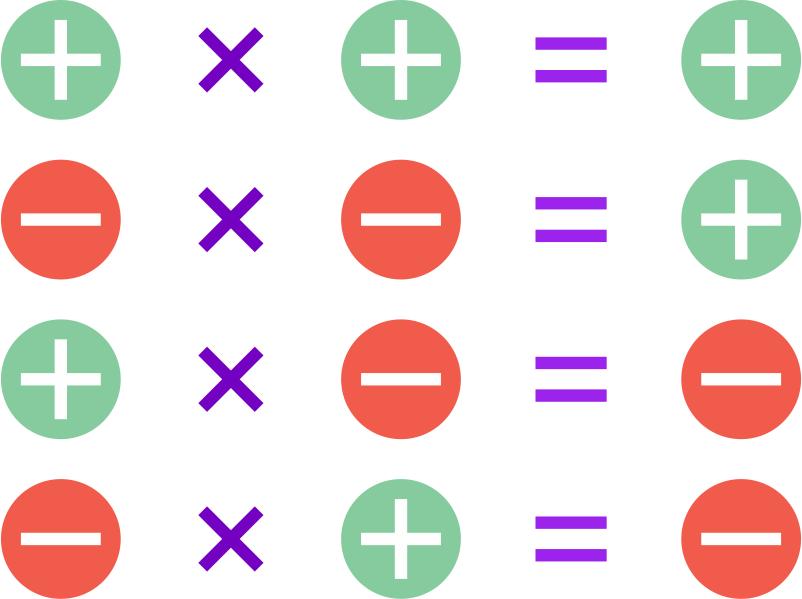The product of integers of the same sign is positive. The product of integers of different signs is negative.

Sign of the product of when more than two integers are multiplied together: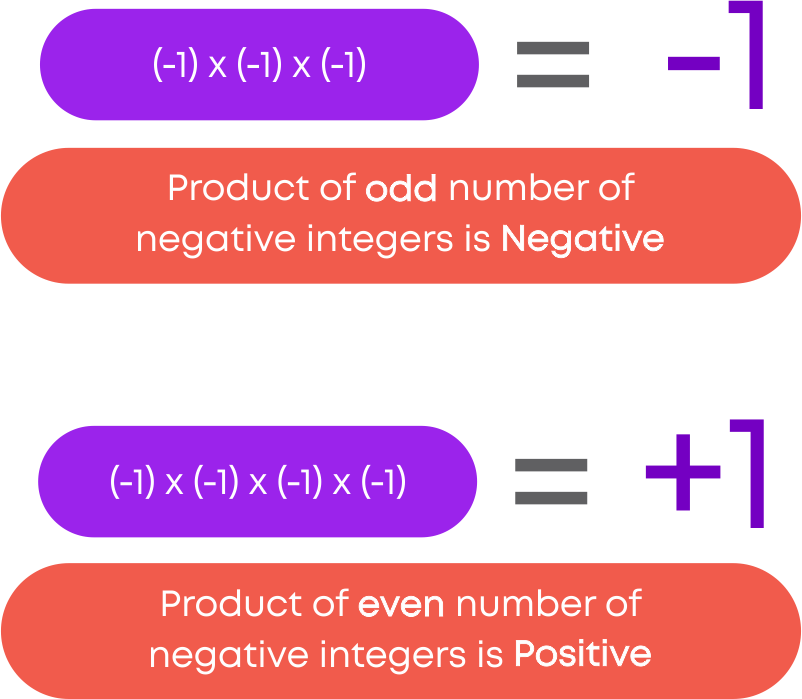While multiplying more than three integers,

• Multiply from the left to the right, taking two at a time
• The product of integers of the same sign is positive
• The product of a positive and a negative integer is negative
• If there are even numbers of negative integers, the product is positive. For example, (-2) × (-2) × (-2) × (-2) = 16
• If there are odd numbers of negative integers, the product is negative. For example, (-2) × (-2) × (-2) × (-2) × (-2) = (-32)

Mathematically, the word difference means

• Less
• The amount reduced

For example, there is an age difference of 5 years between me and my elder sister.

One-third means,

• ‘One by three’
• ‘One of the three equal parts’

For example, Rahim, Sheena and I shared the one-third of the doughnut amongst us. Here, each piece of the doughnut is shared equally.

#### Properties Of Multiplication Of Integers

The product of two integers is always an integer.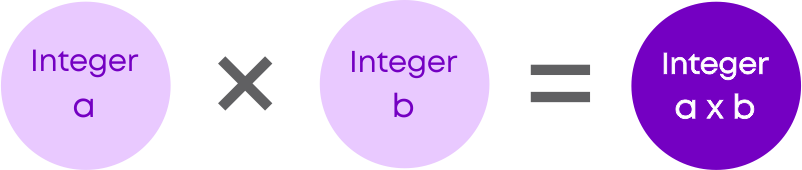We know integers are commutative under addition but are integers commutative under multiplication? Let us multiply two integers (-8) and 10 in two different ways.The product of the integers remains the same even though the integers are ordered differently.

We can say that integers follow commutative property of multiplication.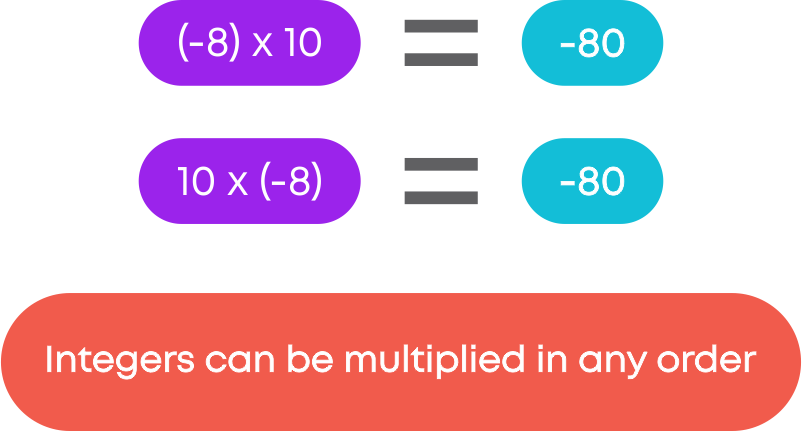Did you know that zero (0) is the only integer that is neither negative nor positive!

The product of any whole number and ‘0’ is ‘0’. The product of any integer and zero is also zero. In general, for any integer ‘a,’

a × 0 = 0 × a = 0

The product of any integer and 1 is the integer itself. In general, for any integer ‘a,’

a × 1 = 1 × a = a

Now, to multiply three integers (-8), (-5) and 10, which two integers should be grouped to multiply first? Are there any rules?The product of the integers remains the same even though the integers are grouped differently.

We can say that integers follow associative property of multiplication.

Associative property of multiplication states that when three integers are multiplied, the product will be the same regardless of the grouping.

In general, if ‘a’, ‘b’ and ‘c’ are three integers then,

(a × b) × c = a × (b × c).

Associative property lets you choose which integers you should multiply first so that multiplication become easier.

Do integers follow distributive property of multiplication over addition? We can verify distributive property using the integers (-8), (-12) and 2.Here the multiplication by (-8) gets distributed over the addition of (-12) and 2.

We can say that integers follow distributive property of multiplication over addition.

Integers follow distributive property of multiplication over subtraction. In general, for three integers, ‘a’, ‘b’ and ‘c’,

a × (b - c) = (a × b) - (a × c)

#### Division Of Integers

When we divide a positive integer by a negative integer, we first divide them as whole numbers and then put a minus sign (–) before the quotient. That is, we get a negative integer.

60 ÷ (-5) = (-12). Hence, the quotient (-12) is negative.

When we divide a negative integer by a positive integer, we divide them as whole numbers and then put a minus sign (–) before the quotient. We, thus, get a negative integer.

(-60) ÷ 5 = (-12). Hence, the quotient (-12) is negative.

When we divide a negative integer by a negative integer, we first divide them as whole numbers and then put a positive sign (+). That is, we get a positive integer.

(-60) ÷ (-5) = 12. Hence, the quotient 12 is positive.

Depending on the signs of the integers that we divide, the sign of the result changes.

Understand these division rules.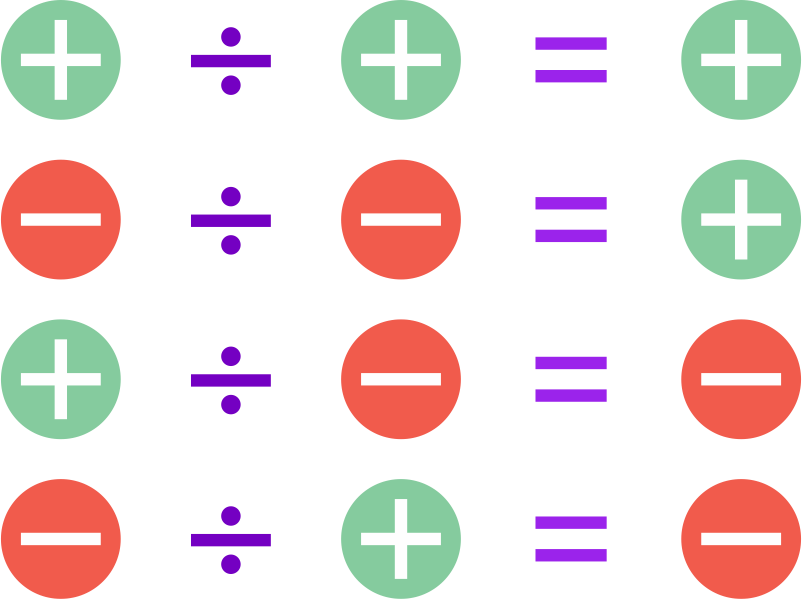Integers are used in many real-life applications. To solve word problems on division of integers, follow these simple points.

• Identify the signs of the numbers in the question whether it is positive or negative
• Identify the operation required to be done in the question
• Carry out the operation to get the final result

A submarine submerges at the rate of 5 m/min. If it descends from 10 m above the sea level, how long will it take to reach 260 m below sea level?

Distance travelled above sea level is denoted with a positive integer while distance travelled below sea level is denoted with a negative integer.

It is given that,

Initial position of the submarine = +10 m

Final position of the submarine = -260 m

Total distance submerged = (-260) – (+10) = -270 m

The submarine submerges at the rate of 5 m/min.

The time taken for 270 m = 270 ÷ 5 = 54 min.

Hence, the submarine will reach 260 m below sea level in 54 minutes.

Product means

• ‘Result ‘
• ‘The answer when 2 numbers are multiplied’

For example, When Anil scored A+ for all subjects, his teacher congratulated him, saying that it was the product of his hard work.

Each means

• ‘Every’
• ‘All’

For example, the teacher said that each student should participate in the Annual Sports day.

Possibility means

• ‘Chances of’

For example, the teacher told the students that there was a possibility for the exams to be postponed if there was a bandh.

#### Properties Of Division Of Integers

Now, you know the properties of integers for addition, subtraction, and multiplication. But does division follow any of the properties? Let us divide ​two integers (-1) and 4 in different ways.

(-1) ÷ 4 = -14

4 ÷ (-1) = (-4)

Hence, division of integers is not commutative.

Also, (-1) ÷ 4 = -14

Here the result of division is not an integer.

Hence, division of integers does not follow closure property.

​Just like whole numbers, division by zero is not defined for integers also. This means we cannot divide an integer by 0. If 0 is divided by any integer, the result is 0.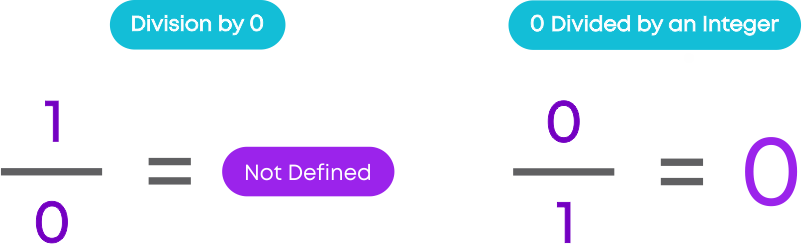You know that any whole number divided by 1 gives the same number as the result.

The same rule applies for integers also.

Any integer divided by 1 gives the same integer as the result. For any integer a, a ÷ 1 = a.

For example, 8 ÷ 1 = 8 and (-18) ÷ 1 = (-18).

#### Common Errors

The following are topics in which students make common mistakes when dealing with integers:

• 1. Sign of integers in multiplication
• 2. Sign of integers in division
• 3. Multiplication of more than two integers

#### Sign Of Integers In Multiplication

Remember the rules of integer multiplication.Make sure that the product has the appropriate sign. For example,

2 × 3 = 6

2 × (-3) = (-6)

(-2) × 3 = (-6)

(-2) × (-3) = 6

#### Sign Of Integers In Division

Remember the rules of integer division.Make sure that the product has the appropriate sign. For example,

10 ÷ 5 = 2

(-10_ ÷ 5 = (-2)

10 ÷ (-5) = (-2)

(-10) ÷ (-5) = 2

#### Multiplication Of More Than Two Integers

While multiplying more than two integers, remember these rules.For example,

2 × 3 × (-1) × (-5) = 30

2 × (-3) × (-1) × (-5) = (-30)

(-2) × (-3) × (-1) × (-5) = 30

2 × 3 × (-1) × 5 = (-30)

#### Conclusion

Integers are everywhere around us. Now that you have learnt about them, can you solve the riddle below?

Observe the pattern and find a, b, c, and d: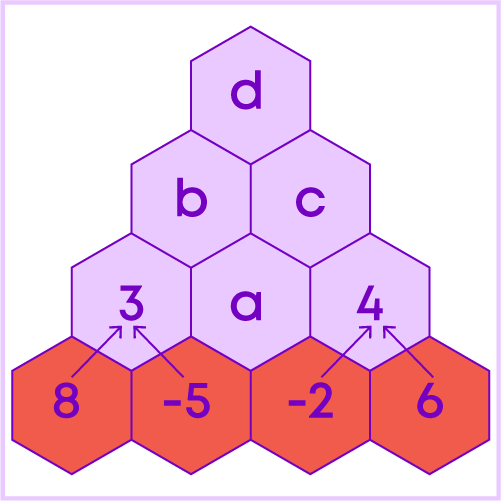#### Jyothi

Author

A math enthusiast and an educationist, Jyothi has a strong academic background and has demonstrated an impressive history of working in the education industry. Jyothi is skilled in developing and reviewing lesson plans, learning content, assessments, and video story boards and scripts.